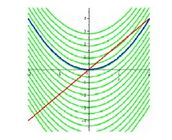Ontario Tech acknowledges the lands and people of the Mississaugas of Scugog Island First Nation.

We are thankful to be welcome on these lands in friendship. The lands we are situated on are covered by the Williams Treaties and are the traditional territory of the Mississaugas, a branch of the greater Anishinaabeg Nation, including Algonquin, Ojibway, Odawa and Pottawatomi. These lands remain home to many Indigenous nations and peoples.

We acknowledge this land out of respect for the Indigenous nations who have cared for Turtle Island, also called North America, from before the arrival of settler peoples until this day. Most importantly, we acknowledge that the history of these lands has been tainted by poor treatment and a lack of friendship with the First Nations who call them home.

This history is something we are all affected by because we are all treaty people in Canada. We all have a shared history to reflect on, and each of us is affected by this history in different ways. Our past defines our present, but if we move forward as friends and allies, then it does not have to define our future.

# Antiderivatives

## Introduction

Derivatives involve finding the rate of change of a function. But what if you were given the rate of change and wanted to know the original function? Well, the process of going from the derivative function to the original is called antidifferentiation; i.e., we find the antiderivative of the given function. Basically, derivatives and antiderivatives undo one another (they are inverse processes).

## WHAT DOES THIS MEAN?

If you’re given a function and asked for its antiderivative, you just have to ask yourself, “I’m looking for a function whose derivative is ____ (given)…what function satisfies that criteria?” Let’s say you want to know the anti-derivative of 2x. You ask yourself, “What function, when I differentiate it, will give me 2x?” Well, x2 satisfies the criteria, so this means that an anti-derivative of 2x is x2.

### THE CONSTANT OF INTEGRATION

Technically, there’s an entire family of functions that are antiderivatives to a given function. Let’s go back to the above example, where we want to find the antiderivative of 2x… sure, x2 satisfies the criteria, but so does x2 + 2 or x2 – 100…all of these have a derivative of 2x because, upon differentiating, the derivative of a constant is 0. So we write that the general antiderivative of 2x is x2 + C, where C is called the constant of integration.

This is illustrated graphically in the figure below, where the red line denotes the function 2x, and the green lines denote the family of antiderivatives, which are of the form x2 + C (parabolas shifted vertically). The blue curve is just an example of an antiderivative (in this case x2).NOTE: If you were given some sort of initial condition, then you could use that to solve for the value of the constant.

### NOTATION

You might see different notation for antiderivatives. Often, we denote the antiderivativeof a function with the same letter, but using a capital. In the example above, we’d say that if f(x) = 2x, then F(x) = x2 and then the most general antiderivative is F(x) + C, which is x2 + C.

Aside:

Alternatively, if you’re taking a calculus class, you might find antiderivatives referred to as “indefinite integrals”, and the notation is then ∫2xdx= x2 + C where the integration sign in front and the “dx” are just part of the notation.

## RULES FOR FINDING ANTIDERIVATIVES

First of all, the following 2 properties (similar to what we had when we found derivatives) are helpful:

• If F(x) is an antiderivative of f(x), then kF(x) is an antiderivative of kf(x).
• If F(x) is an antiderivative of f(x) and G(x) is an antiderivative of g(x), then F(x) ± G(x) is an antiderivative of f(x) ± g(x).

.Additionally, the following table gives several useful antiderivatives.

 f(x) F(x) f(x) F(x) xn (for n≠ -1) xn-1/n+1 sec2x tanx 1/x ln[x] sec x tan x sec x ex ex 1/√1-x2 sin-1x sin x - cos x 1/1+x2 tan-1x cos x sin x

Because differentiation and antidifferentiation “undo” one another, you can check your work by differentiating! i.e., Find the derivative of what you got for F(x), and you should get back the original function in the question, f(x)!!

Example:

Find the antiderivative of $f(x)= x^2 – 6x +1$

Solution:

$$F(x) = \frac{1}{3}x^3 – 3x^2 + x + C$$

Example:

Find the antiderivative of $f(x) = 7cos(x) – \frac{2}{x} + 9e^x$

Solution:

$$F(x) = 7\sin{x} – 2\ln{|x|} + 9e^x + C$$

Example:

Find the antiderivative of  $$f(x) = \frac{1}{x^3} + \sqrt{x} + 8$$.

Solution:

$$\displaylines{f(x) = x^{-3} +x^{1/2} + 8 \\ F(x) = -\frac{1}{2}x^{-2} + \frac{2}{3}x^{3/2} + 8x + C}$$

Example 1:

Example 2: